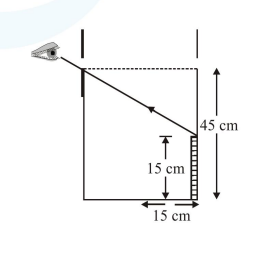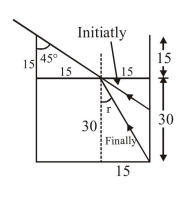# An observer can see through a small hole on the side

Question:

An observer can see through a small hole on the side of a jar (radius $15 \mathrm{~cm}$ ) at a point at height of $15 \mathrm{~cm}$ from the bottom (see figure). The hole is at a height of $45 \mathrm{~cm}$. When the jar is filled with a liquid up to a height of $30 \mathrm{~cm}$ the same observer can see the edge at the bottom of the jar. If the refractive index of the liquid $\mathrm{N} / 100$, where $\mathrm{N}$ is an integer, the value of $\mathrm{N}$ is__________.Solution:$\tan \mathrm{r}=\frac{15}{30}=\frac{1}{2}$

$\sin r=\frac{1}{\sqrt{5}}$

$1 \sin 45^{\circ}=\mu \sin \mathrm{r}$

$\frac{1}{\sqrt{2}}=\mu\left(\frac{1}{\sqrt{5}}\right)$

$\mu=\sqrt{\frac{5}{2}}=1.581$

$\frac{\mathrm{N}}{100}=\mu$

$N=100 \mu$

$N=158.11$

So integer value of $\mathrm{N}=15800$